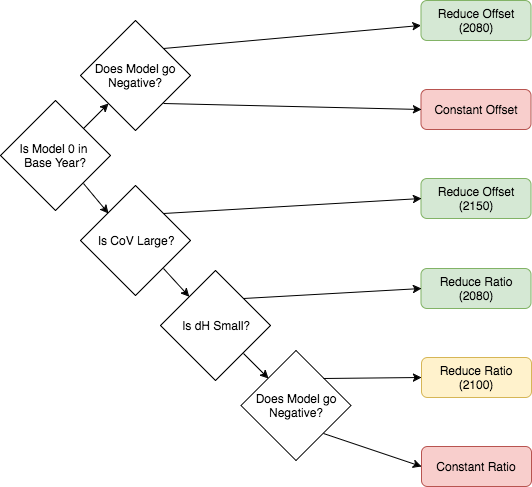# Theory¶

## Methods¶

All harmonization is based on the following equations.

$$\beta$$: the harmonization convergence parameter

$\begin{split}\begin{equation}\label{eqs:factor} \beta(t, t_i, t_f) = \begin{cases} 1 - \frac{t - t_i}{t_f - t_i},& \text{if } t \leq t_f\\ 0, & \text{otherwise} \end{cases} \end{equation}\end{split}$

$$m^{rat}$$: ratio-based harmoniation

$\begin{equation}\label{eqs:ratio} m^{rat}(t, m, h, t_i, t_f) = [\beta(t, t_i, t_f) (\frac{h(t_i)}{m(t_i)} - 1) + 1] m(t) \end{equation}$

$$m^{off}$$: offset-based harmoniation

$\begin{equation}\label{eqs:offset} m^{off}(t, m, h, t_i, t_f) = \beta(t, t_i, t_f) (h(t_i) - m(t_i)) + m(t) \end{equation}$

$$m^{int}$$: linear-interoplation-based harmoniation

$\begin{split}\begin{equation}\label{eqs:interpolate} m^{int}(t, m, h, t_i, t_f) = \begin{cases} \frac{m(t_f) - h(t_i)}{t_f - t_i}(t - t_i) + h(t_i), & \text{if } t \leq t_f\\ m(t), & \text{otherwise} \end{cases} \end{equation}\end{split}$

These harmonization methods are made available in aneris by name selection. Available names are listed below:

All Harmonization Methods Provided in aneris
Method Name Harmonization Family Convergence Year
constant_ratio ratio $$t_f = \infty$$
reduce_ratio_<year> ratio $$t_f = \texttt{<year>}$$
constant_offset offset $$t_f = \infty$$
reduce_offset_<year> offset $$t_f = \texttt{<year>}$$
linear_inerpolate_<year> interpolation $$t_f = \texttt{<year>}$$

## Default Decision Tree¶

While any method can be used to harmonize a given trajectory, intelligent defaults are made available to the user. These default methods are deteremined by use of a decision tree, which analyzes the historical trajectory, model trajectory, and relative difference between trajectories in the harmonization year. The decision tree as implemented is provided below:The decision tree use in aneris to determine default methods to use for each trajectory.